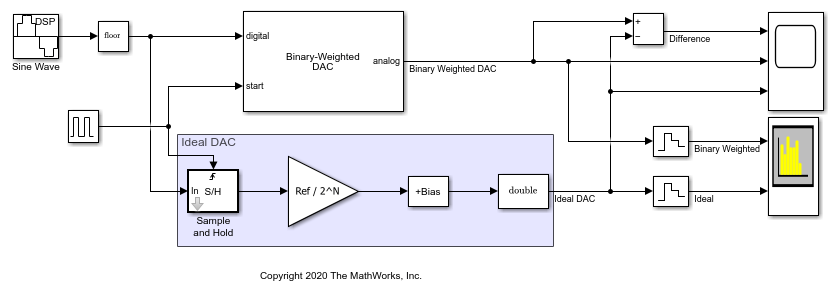# Compare Binary Weighted DAC to Ideal DAC

This example shows a comparison of the Binary Weighted DAC from the Mixed-Signal Blockset™ to an ideal DAC model.

This model compares the Binary Weighted DAC and an equivalent ideal DAC model. For the comparison to be valid, both DACs must sample their inputs concurrently. The parameters (reference, bias, sampling frequency, number of bits) of both the Binary Weighted DAC and the ideal DAC are derived from the TLC5615 datasheet.

The ideal DAC model is composed of a Sample and Hold block, Gain block, Bias block and Data Type Conversion block. The Sample and Hold ensures input data enters the DAC at the correct rate (`Fs`) even if the digital input signal was sampled at some other rate. The Gain block scales the digital word to an analog scale and the Bias block applies a bias to match the output of the Binary Weighted DAC. The Binary Weighted DAC uses its reference parameter as its full scale output range, thereby making its throughput gain equivalent to `Ref / (2^NBits - 1)`. The Data Type Conversion block ensures the output data type matches the Binary Weighted DAC.

The Binary Weighted DAC outputs a scalar double. The outputs of both the Binary Weighted DAC and the ideal DAC produce values on the interval `[-Ref, Ref - 1 LSB] / 2`.

```model = 'BinDacIdealCompare'; open_system(model); ```The Scope block shows the difference between the two outputs on the left and the two outputs themselves on the right.

```open_system([model '/Time Scope']); sim(model); ```The Spectrum Analyzer shows the spectra of the two outputs. The Spectrum Analyzer requires fixed-step sampled inputs, which the Zero-Order Hold blocks perform at the same rate that the DAC performs conversions, `Fs`.

```close_system([model '/Time Scope']); open_system([model '/Spectrum Analyzer']); sim(model); ```